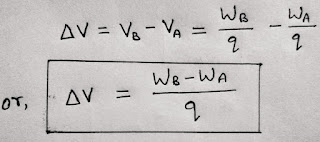# Electric Potential Difference by A.K.Sinha

Electric Potential difference :

Video lecture 👇👇👇

The electric potential difference between two points in an electric field is defined as the difference in work done in bringing a unit positive charge from infinity to that two different points.

We can also define it as the work done to move an unit positive charge between two points in an electrostatic field.

CONCEPT :

We consider two points A & B in an electric field as shown in fig.

We consider an unit positive charge at infinity. Now we move that charge from infinity to a point A in an electrostatic field . Let the work done is WA . Again if we move the same charge from infinity to any other point B in same field then the work done is WB.

These work done are the electric potential of point A and B respectively as the charge is unit.

Hence,

VA = WA

&.           VB = WB

Hence the difference in Electric potential between two points is

∆V = VB - VA = WB - WA

Now, if the charge at infinity is q , then we can rewrite the above equation for Electric potential difference asAbove expression is the required expression for Electric potential difference.

The SI unit of electric potential difference is same as of electric potential ( i.e. volt or V ) because it is the difference between Electric potential of two points.